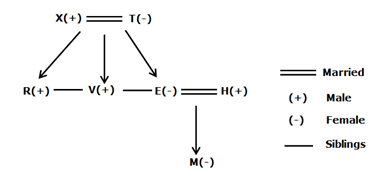# SBI Clerk Prelims Reasoning Ability Questions 2021 (Day-46)

Dear Aspirants, Our IBPS Guide team is providing new series of Reasoning Questions for SBI Clerk Prelims 2021 so the aspirants can practice it on a daily basis. These questions are framed by our skilled experts after understanding your needs thoroughly. Aspirants can practice these new series questions daily to familiarize with the exact exam pattern and make your preparation effective.

Start Quiz

Blood relation

Direction (1-2): Study the following information carefully and answer the below questions.

A family consists of seven members and two married couples. E is the only daughter of T who is the mother of V. H is the brother-in-law of R. M is the niece of R. X and T are a married couple. H and R are the same genders. H is the son-in-law of X.

1) How V is related to M?

A.Uncle

B.Aunty

C.Brother-in-law

D.Sister-in-law

E.Can’t be determined

2) Who among the following is the brother of E?

A.V

B.R

C.H

D.Both a and b

E.Both b and c

Number series

Direction (3-7): Read the following information carefully and answer the questions given below.

6 5 8 9 6 2 4 5 3 6 2 1 5 2 3 6 5 8 6 2 4 5 6 3 6 4 2

3) Which of the following number is exactly between the third odd number from the right end and the fifth even number fromthe left end?

A.1

B.3

C.2

D.5

E.None

4) How many such odd numbers are there each of which is immediately preceded and immediately followed by an even number?

A.Three

B.Four

C.Six

D.Five

E.More than six

5) Which of the following is the next pair in the series(the pair starts from the left end)?

689, 253, 252___

A.358

B.658

C.686

D.524

E.358

6) How many numbers are there between the eighth number from the left end and the tenth number from the right end?

A.Six

B.Five

C.Eight

D.Nine

E.Seven

7) If in the given number “2153674562”,1 is subtracted from the odd digit and 2 is added to the even digit of the number, then which of the following digit(s) are repeated more than three times?

A.8

B.4

C.6

D.0

E.2

Inequality

Direction (8-10): In each of the following questions, the relationship between different elements is shown in the statements followed by two conclusions. Find a true conclusion.

8) Statements:

J < M < Q ≤I = K ≤ V > S = U > A

Conclusions:

I). Q ≤ V

II). V ≥ A

A.If only conclusion I follows.

B.If only conclusion II follows.

C.If either conclusion I or II follows.

D.If neither conclusion I nor II follows.

E.If both conclusions I and II follow.

9) Statements:

Z > R > T = Y ≥ M < Q≥ S ≤ I = H

Conclusions:

I). M ≤ Z

II). H < Y

A.If only conclusion I follows.

B.If only conclusion II follows.

C.If either conclusion I or II follows.

D.If neither conclusion I nor II follows.

E.If both conclusions I and II follow.

10) Statements:

H ≤ Q ≤ T < Y < A = D ≥ I≥ C ≥ M = B

Conclusions:

I). B ≤ A

II). H < D

A.If only conclusion I follows.

B.If only conclusion II follows.

C.If either conclusion I or II follows.

D.If neither conclusion I nor II follows.

E.If both conclusions I and II follow.

Directions (1-2) :Given series

6 5 8 9 6 2 4 5 3 6 2 1 5 2 3 6 5 8 6 2 4 5 6 3 6 4 2

Given series

6 5 8 9 6 2 4 5 3 6 2 1 5 2 3 6 5 8 6 2 4 5 6 3 6 4 2

Required arrangement: Even number -> odd number -> even numtber

6 5 8 9 6 2 4 5 3 6 2 1 5 2 3 6 5 8 6 2 4 5 63 6 4 2

Given series

6 5 8 9 6 2 4 5 3 6 2 1 5 2 3 6 5 8 6 2 4 5 6 3 6 4 2

Given series

6 5 8 9 6 2 4 3 6 2 1 5 2 3 6 5 8 6 2 4 5 6 3 6 4 2

3 6 2 1 5 2 3 6 5

2153674562

4042866484

I). Q ≤ V (Q ≤ I = K ≤ V) -> True

II). V ≥ A ( V > S= U > A) -> False

Only conclusion I follows.

I). M ≤ Z ( Z > R > T = Y ≥ M ) -> False

II). H < Y ( Y ≥ M < Q ≥ S ≤ I = H) -> False

Neither conclusion I nor II follows

I). B ≤ A (A = D ≥ I ≥ C ≥ M = B ) -> True

II). H < D (H ≤ Q ≤ T < Y < A = D ) -> True

Both conclusions I and II follow

 Check Here to View SBI Clerk Prelims 2021 Reasoning Ability Questions Day – 45 Day – 44 Day – 43